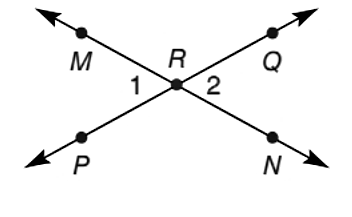Chapter 1.2, Problem 16E### Elementary Geometry for College St...

6th Edition
Daniel C. Alexander + 1 other
ISBN: 9781285195698

#### Solutions

Chapter
Section### Elementary Geometry for College St...

6th Edition
Daniel C. Alexander + 1 other
ISBN: 9781285195698
Textbook Problem
1 views

# When two lines cross (intersect), they have exactly one point in common. In the drawing, what is the point of intersection? How do the measure of ∠ 1 and ∠ 2 compare?To determine

To find:

The point of intersection and to compare the measures of 1 and 2.

Explanation

Calculation:

Given,

The point R is the common point for both the lines MN and PQ.

Thus, R is the point of intersection

### Still sussing out bartleby?

Check out a sample textbook solution.

See a sample solution

#### The Solution to Your Study Problems

Bartleby provides explanations to thousands of textbook problems written by our experts, many with advanced degrees!

Get Started

#### Find more solutions based on key concepts